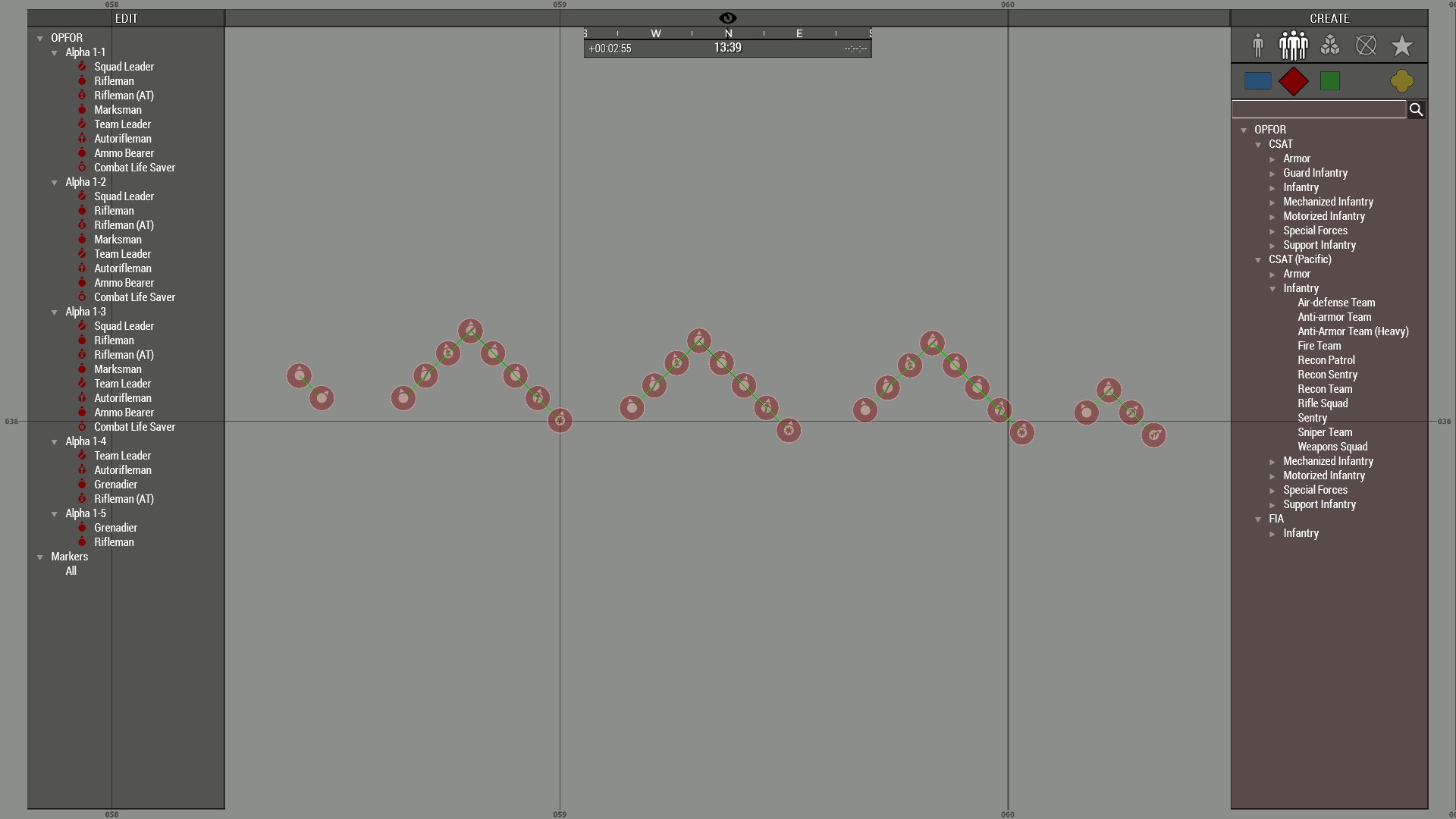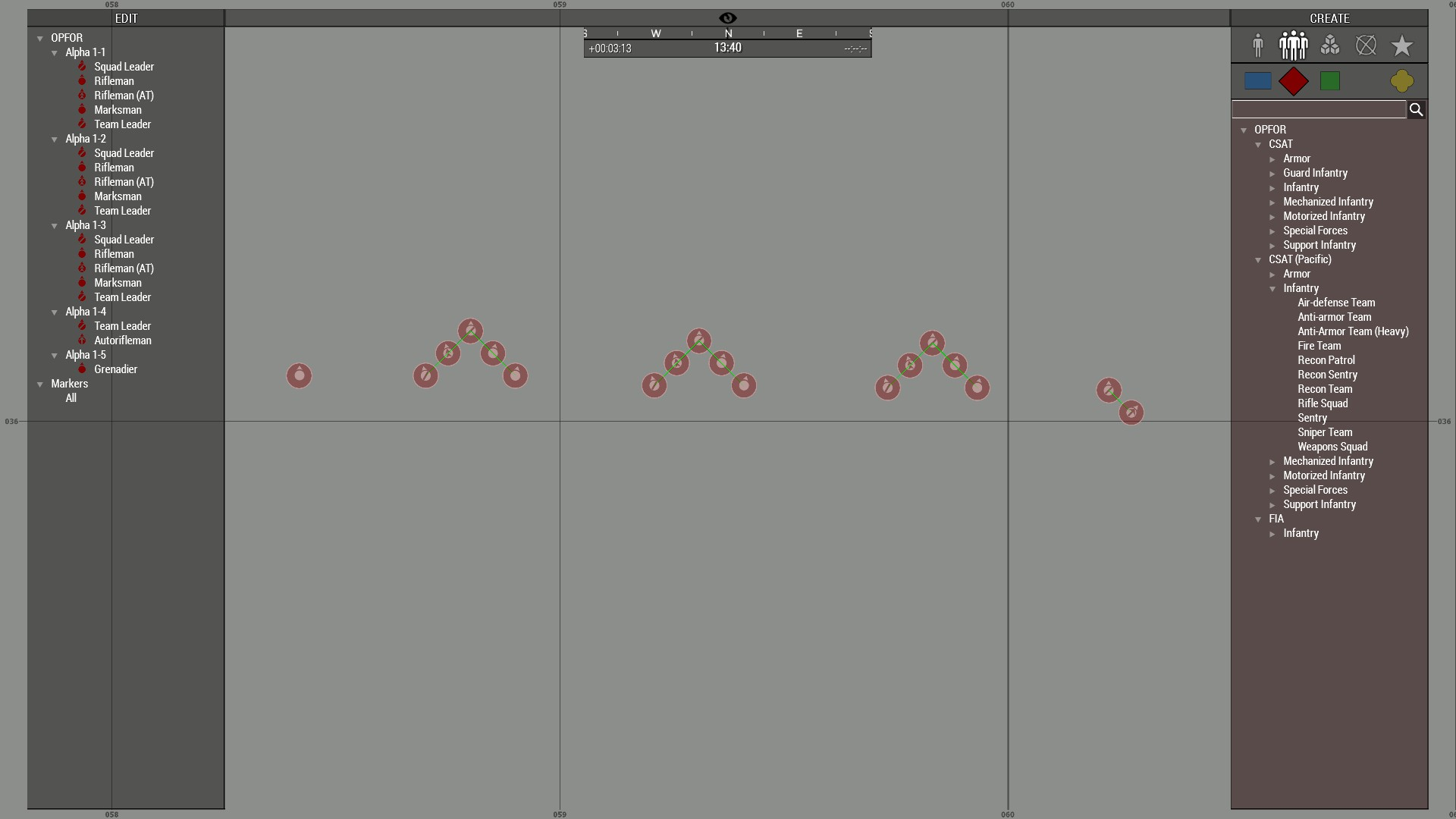## Recommended Posts

Hello!

Really sorry If offtop, but I have a question.

Now I make my own cooperative mission and wanna delete/add squads according players, so, there is two sub-question:
1) How I can check players count?
2) How I can delete already installed on map squads of AI?

Thanks!

##### Share on other sites

a method i like to use is not to delete entire groups, but to trim them, so perhaps they have 5-6 units instead of 8.

heres an example:

default number of unitstrimmed number of unitsand a block of code to achieve something like that could look like this:

```// init.sqf
if (isServer) then {
_maxplayers = 10;
_playerCount = count allPlayers;
_multiplier = _playerCount / _maxplayers;	// example:   _multiplier = 4 / 10;
private _grp = grpnull;
private _grpcount = -1;
private _newcount = -1;
{
if ((side _x) in [EAST]) then {
_grp = _x;
_grpcount = count (units _grp);
_newcount = round (_grpcount * _multiplier);
while {((count (units _grp)) > _newcount)} do {
deleteVehicle ((units _grp) select ((count (units _grp)) - 1));
};
};
} forEach allGroups;
};```

•2